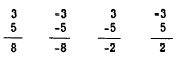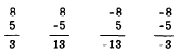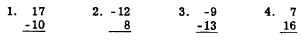Operating with signed numbersCustom SearchOPERATING WITH SIGNED NUMBERS The number line can be used to demonstrate addition of signed numbers. Two cases must be considered; namely, adding numbers with like signs and adding numbers with unlike signs. ADDING WITH LIKE SIGNS As an example of addition with like signs, suppose that we use the number line (fig. 3-4) to add 2 + 3. Since these are signed numbers, we indicate this addition as (+2) + (+3). This emphasizes that, among the three + signs shown, two are number signs and one is a sign ofFigure 3-4.-Using the number line to add. operation. Line a (fig. 3-4) above the number line shows this addition. Find 2 on the number line. To add 3 to it, go three units more in a positive direction and get 5. To add two negative numbers on the number line, such as -2 and -3, find -2 on the number line and then go three units more in the negative direction to get -5, as in b (fig. 3-4) above the number line. Observation of the results of the foregoing operations on the number line leads us to the following conclusion, which may be stated as a law: To add numbers with like signs, add the absolute values and prefix the common sign. ADDING WITH UNLIKE SIGNS To add a positive and a negative number, such as (-4) + (+5), find +5 on the number line and go four units in a negative direction, as in line c above the number line in figure 3-4. Notice that this addition could be performed in the other direction. That is, we could start at -4 and move 5 units in the positive direction. (See line d, fig. 3-4.) The results of our operations with mixed signs on the number line lead to the following conclusion, which may be stated as a law: To add numbers with unlike signs, find the difference between their absolute values and prefix the. sign of the numerically greater number. The following examples show the addition of the numbers 3 and 5 with the four possible combinations of signs:In the first example, 3 and 5 have like signs and the common sign is understood to be positive. The sum of the absolute values is 8 and no sign is prefixed to this sum, thus signifying that the sign of the 8 is understood to be positive. In the second example, the 3 and 5 again have like signs, but their common sign is negative. The sum of the absolute values is 8, and this time the common sign is prefixed to the sum. The answer is thus -8. In the third example, the 3 and 5 have unlike signs. The difference between their absolute values is 2, and the sign of the larger addend is negative. Therefore, the answer is -2. In the fourth example, the 3 and 5 again have unlike signs. The difference of the absolute values is still 2, but this time the sign of the larger addend is positive. Therefore, the sign prefixed to the 2 is positive (understood) and the final answer is simply 2. These four examples could be written in a different form, emphasizing the distinction between the sign of a number and an operational sign, as follows: (+3) + (+5) = +8 (-3) + (-5) = -8 (+3) + (-5) = -2 (-3) + (+5) = +2 Practice problems. Add as indicated: 1. -10 + 5 = (-10) + (+5) = ? 2. Add -9, -16, and 25 3. -7 -1 - 3 = (-7) + (-1) + (-3) = ? 4. Add -22 and -13 Answers: 1.   -5 2.   -0 3.  -11 4.  -35 SUBTRACTION Subtraction is the inverse of addition. When subtraction is performed, we "take away" the subtrahend. This means that whatever the value of the subtrahend, its effect is to be reversed when subtraction is indicated. In addition, the sum of 5 and -2 is 3. In subtraction, however, to take away the effect of the -2, the quantity +2 must be added. Thus the difference between +5 and -2 is +7. Keeping this idea in mind, we may now proceed to examine the various combinations of subtraction involving signed numbers. Let us first consider the four possibilities where the minuend is numerically greater than the subtrahend, as in the following examples:We may show how each of these results is obtained by use of the number line, as shown in figure 3-5. In the first example, we find +8 on the number line, then subtract 5 by making a movement that reverses its sign. Thus, we move to the left 5 units. The result (difference) is +3. (See line a, fig. 3- 5 .) In the second example, we find +8 on the number line, then subtract (-5) by making a movement that will reverse its sign. Thus we move to the right 5 units. The result in this case is +13. (See line b, fig. 3-5.) In the third example, we find -8 on the number line, then subtract 5 by making a movement that reverses its sign. Thus we move to the left 5 units. The result is -13. (See line c, fig. 3-5.) In the fourth example, we find -8 on the number line, then reverse the sign of -5 by moving 5 units to the right. The result is -3. (See line d, fig. 3-5.) Next, let us consider the four possibilities that arise when the subtrahend is numerically greater than the minuend, as in the following examples:In the first example, we find +5 on the number line, then subtract 8 by making a movementFigure 3-5.-Subtraction by use of the number line. that reverses its sign. Thus we move to the left 8 units. The result is -3. (See line e, fig. 3-5.) In the second example, we find +5 on the number line, then subtract -8 by making a movement to the right that reverses its sign. The result is 13. (See line f, fig. 3-5.) In the third example, we find - 5 on the number line, then reverse the sign of 8 by a movement to the left. The result is -13. (See line g, fig. 3-5.) In the fourth example, we find -5 on the number line, then reverse the sign of -8 by a movement to the right. The result is 3. (See line h, fig. 3-5.) Careful study of the preceding examples leads to the following conclusion, which is stated as a law for subtraction of signed numbers: In any subtraction problem, mentally change the sign of the subtrahend and proceed as in addition. Practice problems. In problems 1 through 4, subtract the lower number from the upper. In 5 through 8, subtract as indicated.5. 1 -(-5) = ? 6. -6 -(-8) = ? 7. 14 - 7 -(-3) = ? 8. -9 - 2 = ? Answers: 1. 27  2. -20  3. 4  4. -9 5. 6  6. 2  7. 10  8. -11Integrated Publishing, Inc. - A (SDVOSB) Service Disabled Veteran Owned Small Business